# Selina Solutions Concise Maths Class 7 Chapter 16: Pythagoras Theorem

Selina Solutions Concise Maths Class 7 Chapter 16 Pythagoras Theorem are prepared by subject matter experts to help students ace the exam. Pythagoras Theorem states that, “In a right angled triangle, the square on the hypotenuse is equal to the sum of the squares on the other two sides”. This is one of the important concepts, which will also be continued in higher classes. Selina Solutions Concise Maths Class 7 Chapter 16 Pythagoras Theorem, PDF links are given here for free download.

Chapter 16 explains the concept of Pythagoras Theorem and its converse, as per the latest syllabus of the ICSE board. The numerous solved examples in the textbook improves logical and analytical thinking abilities among students.

## Selina Solutions Concise Maths Class 7 Chapter 16: Pythagoras Theorem Download PDF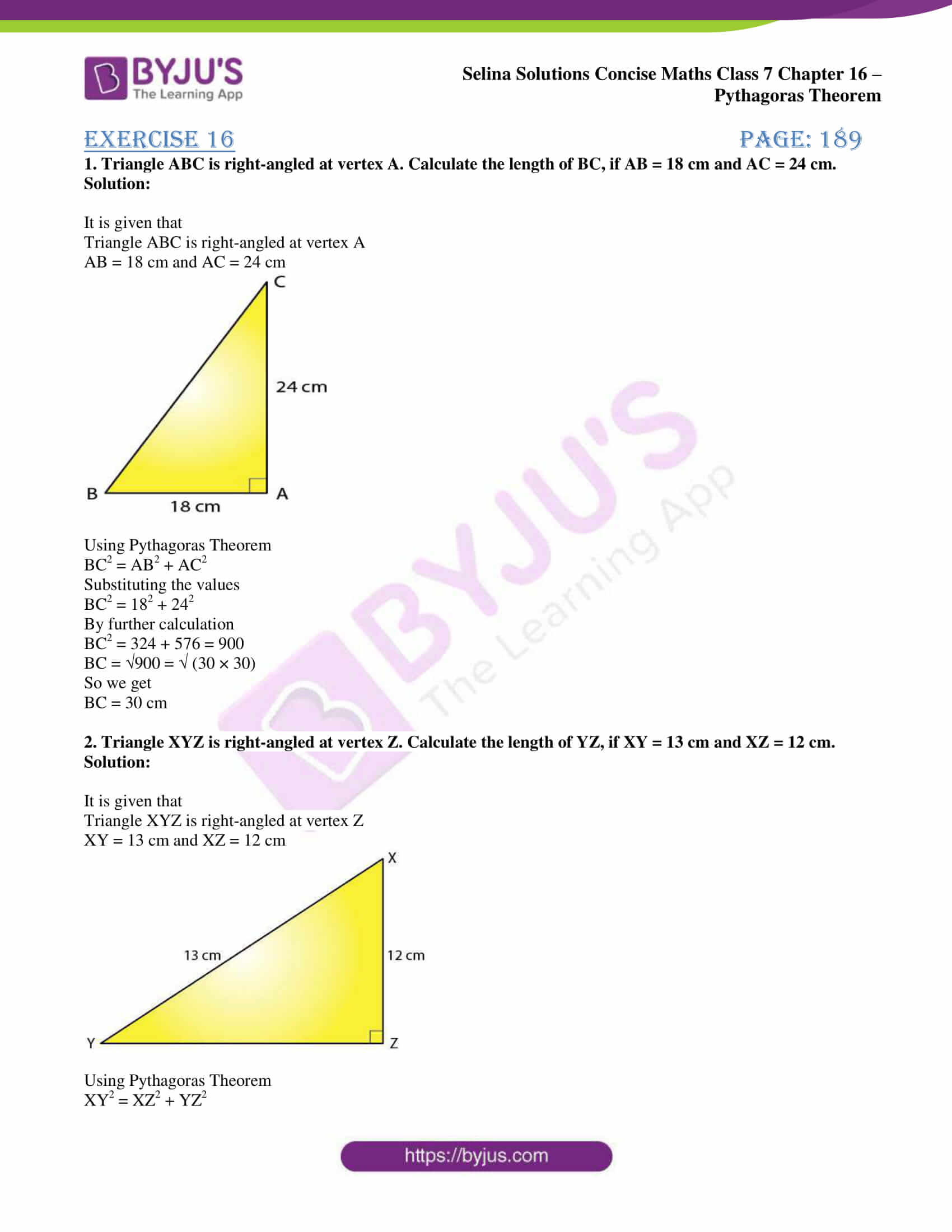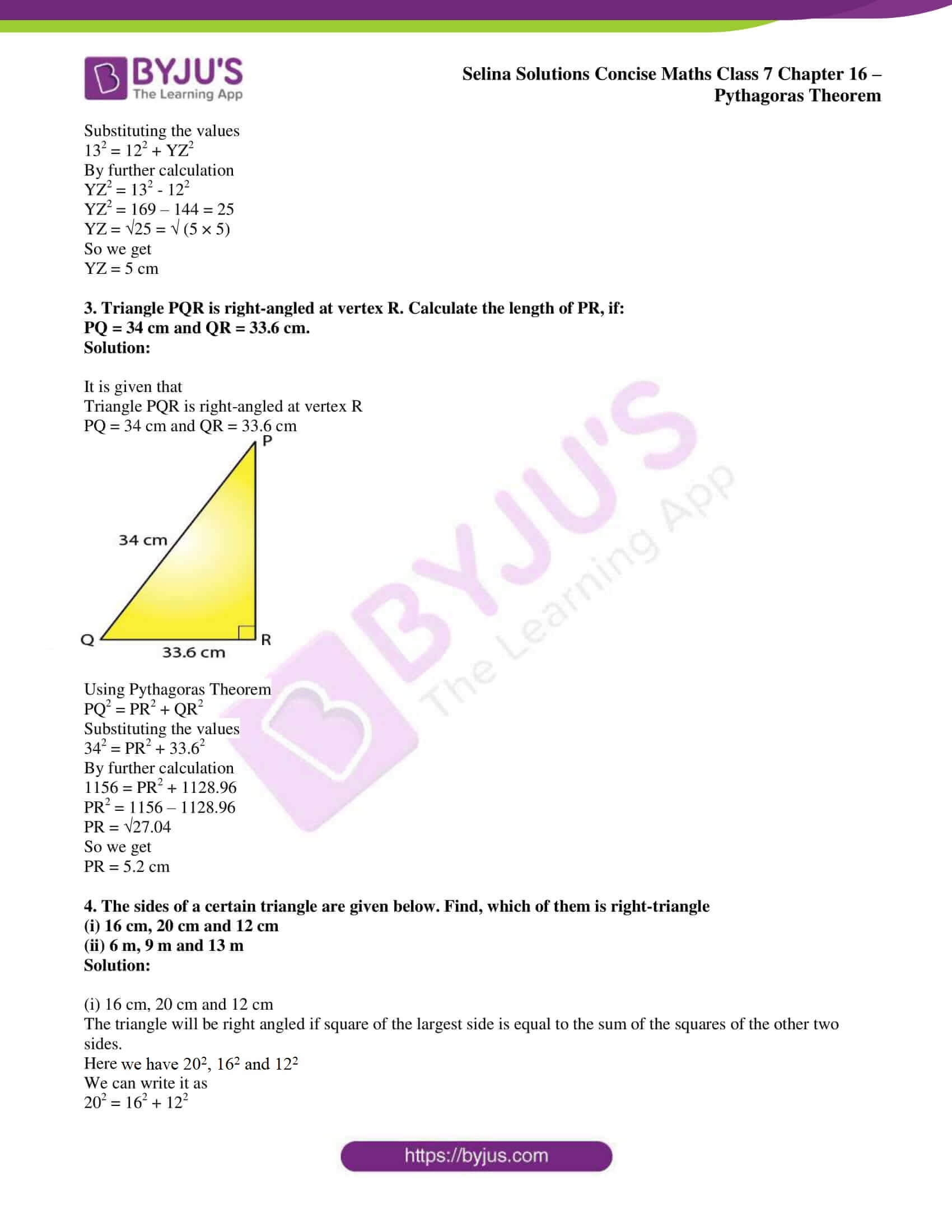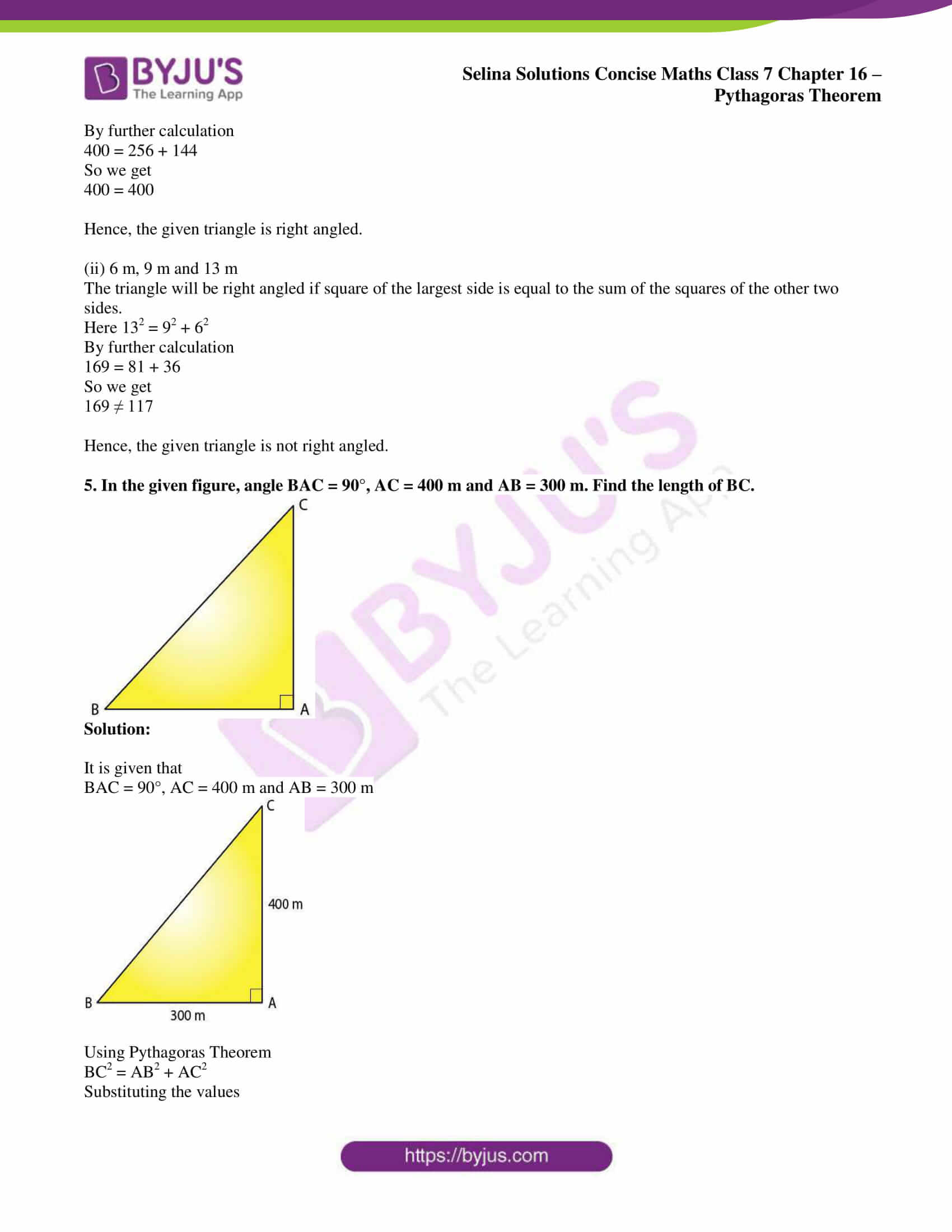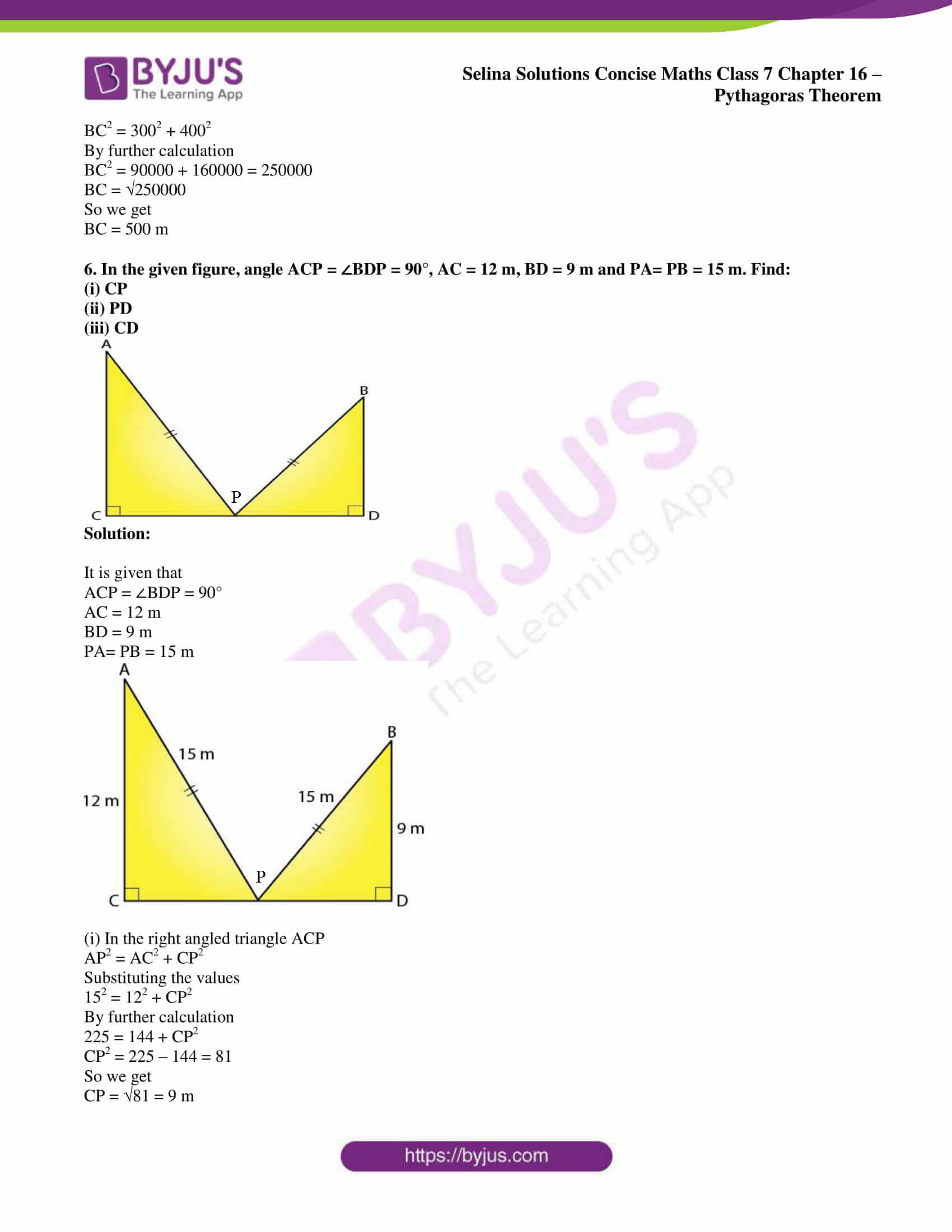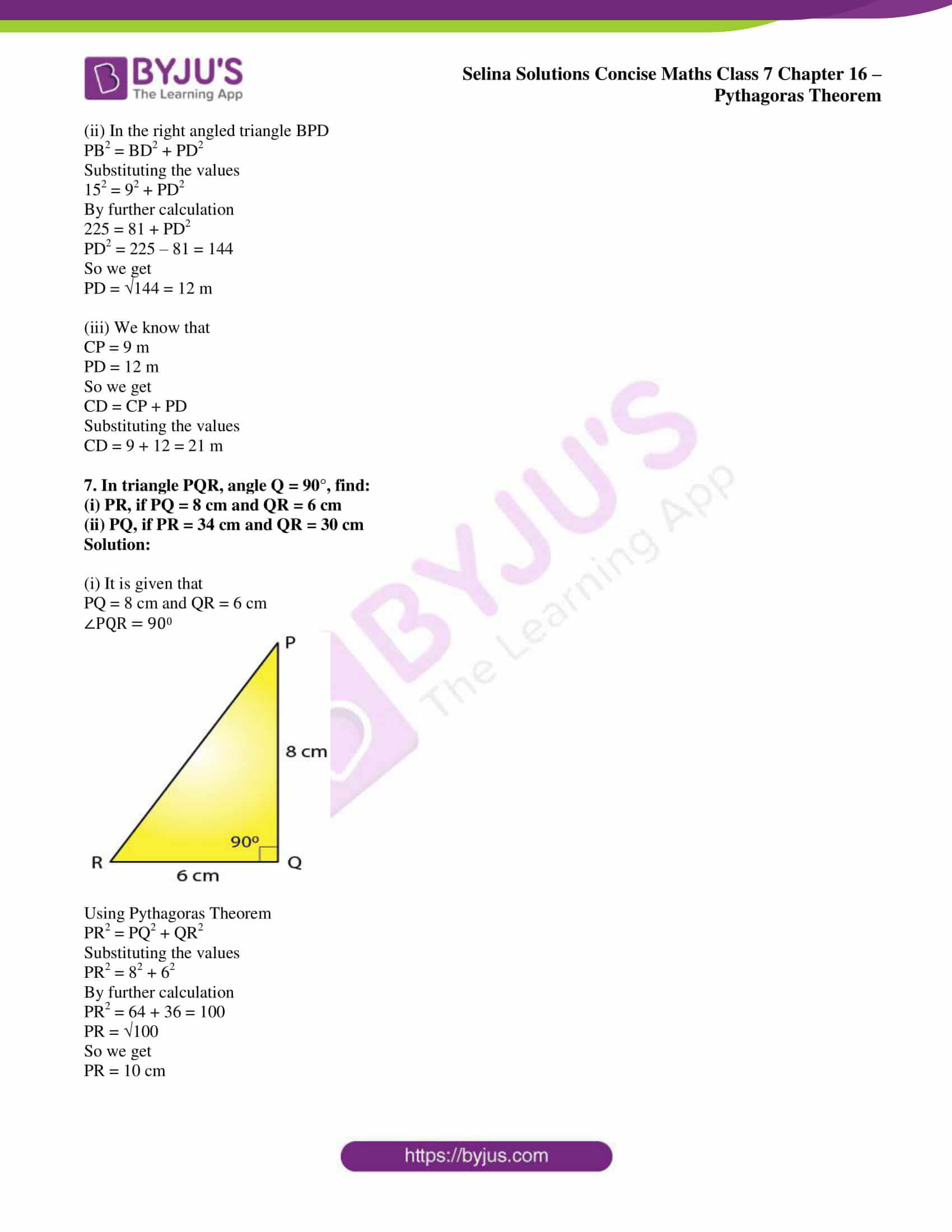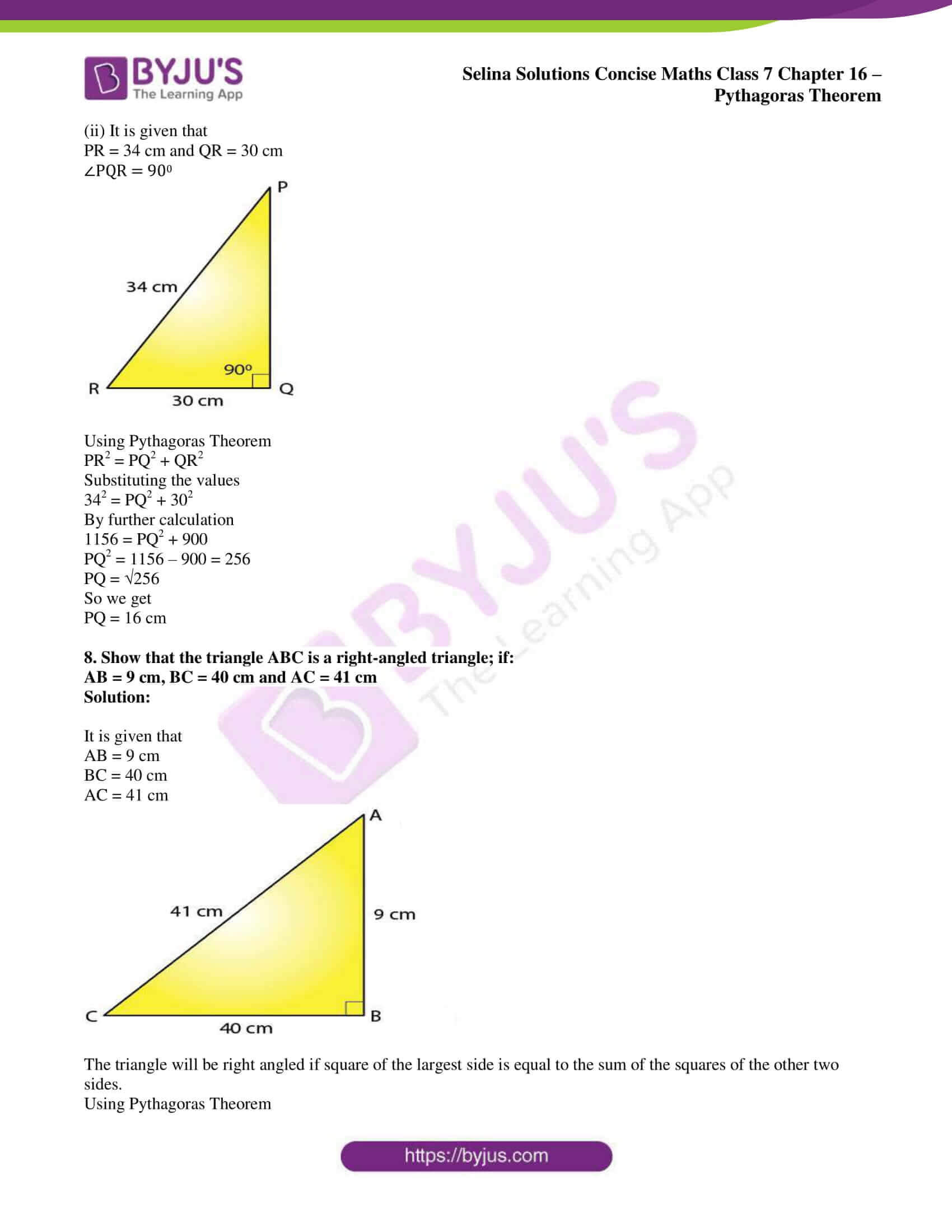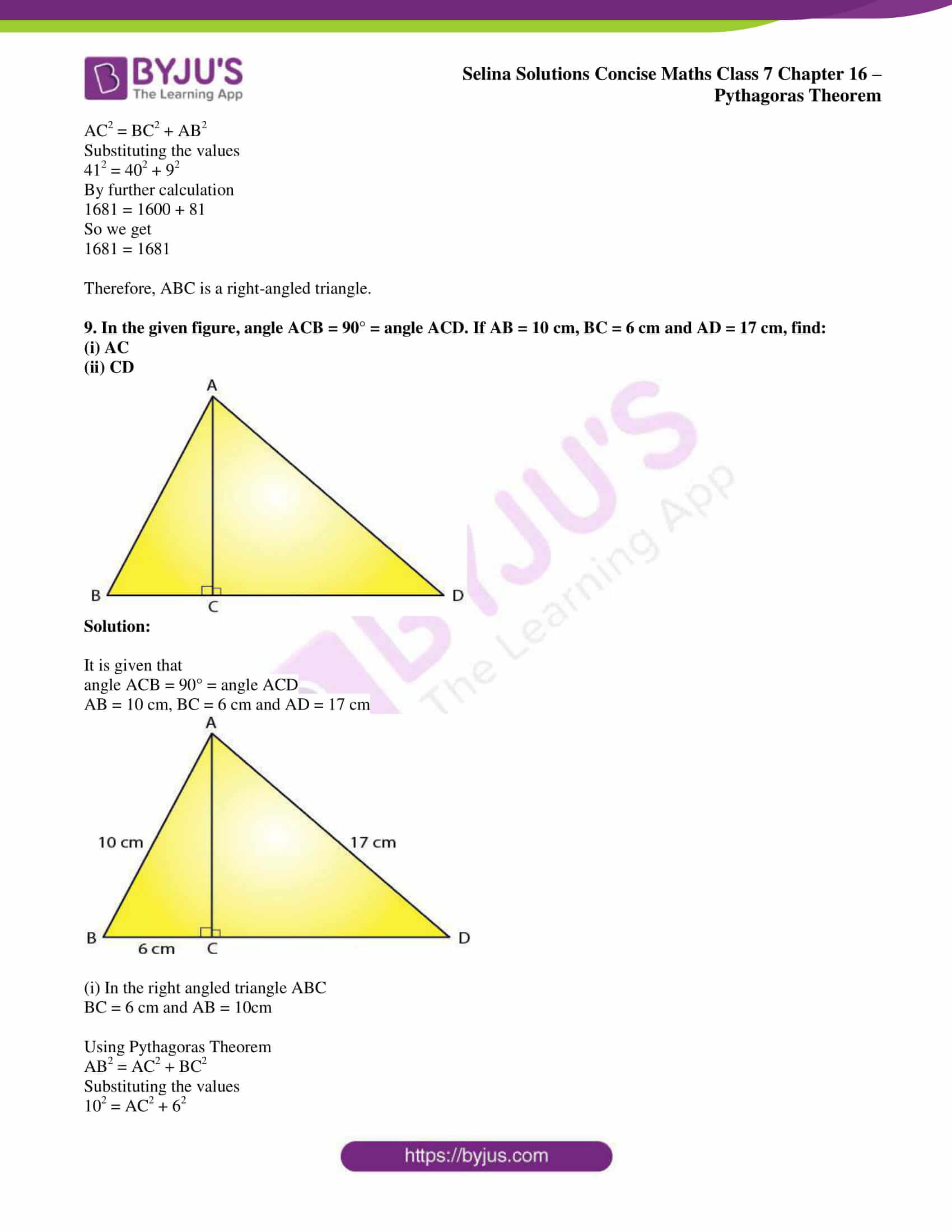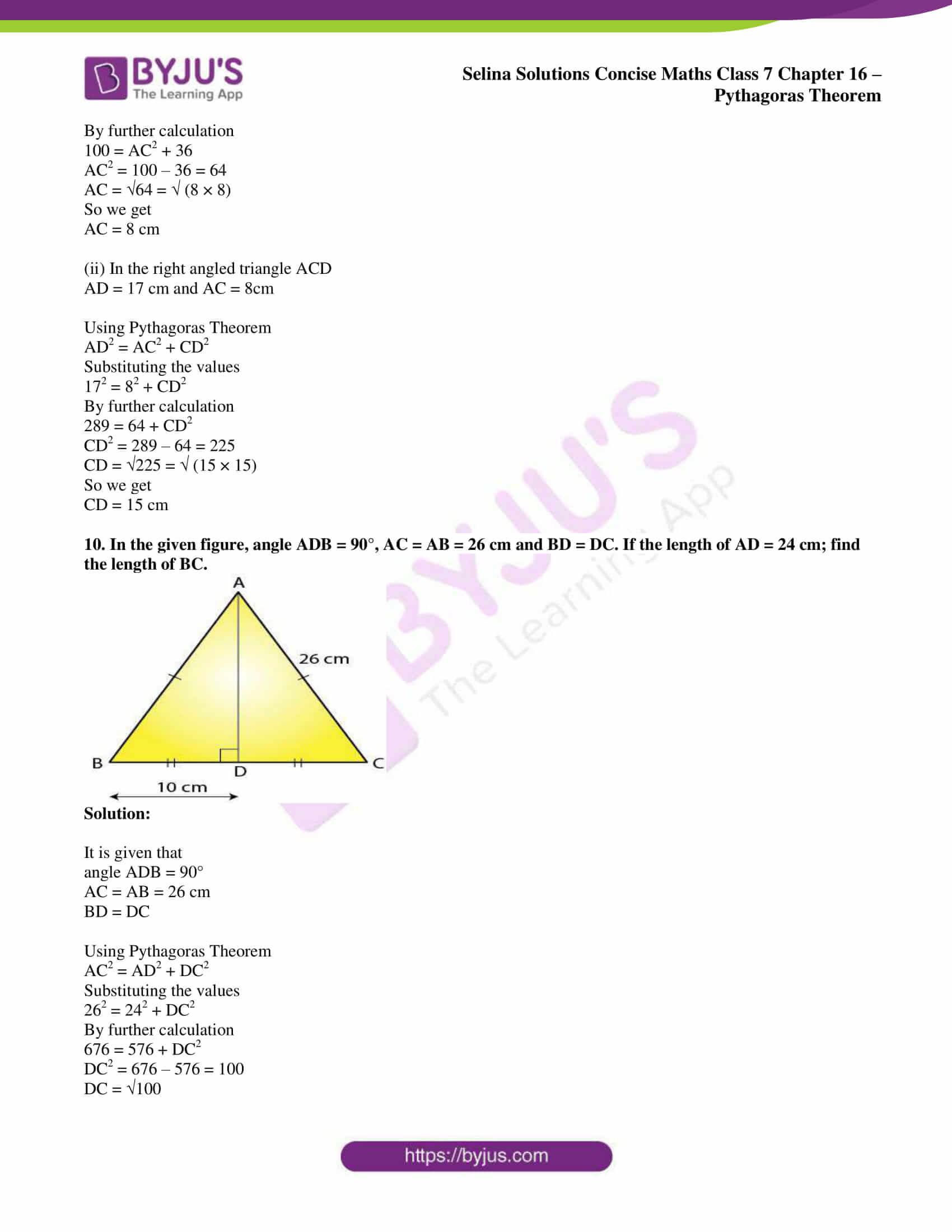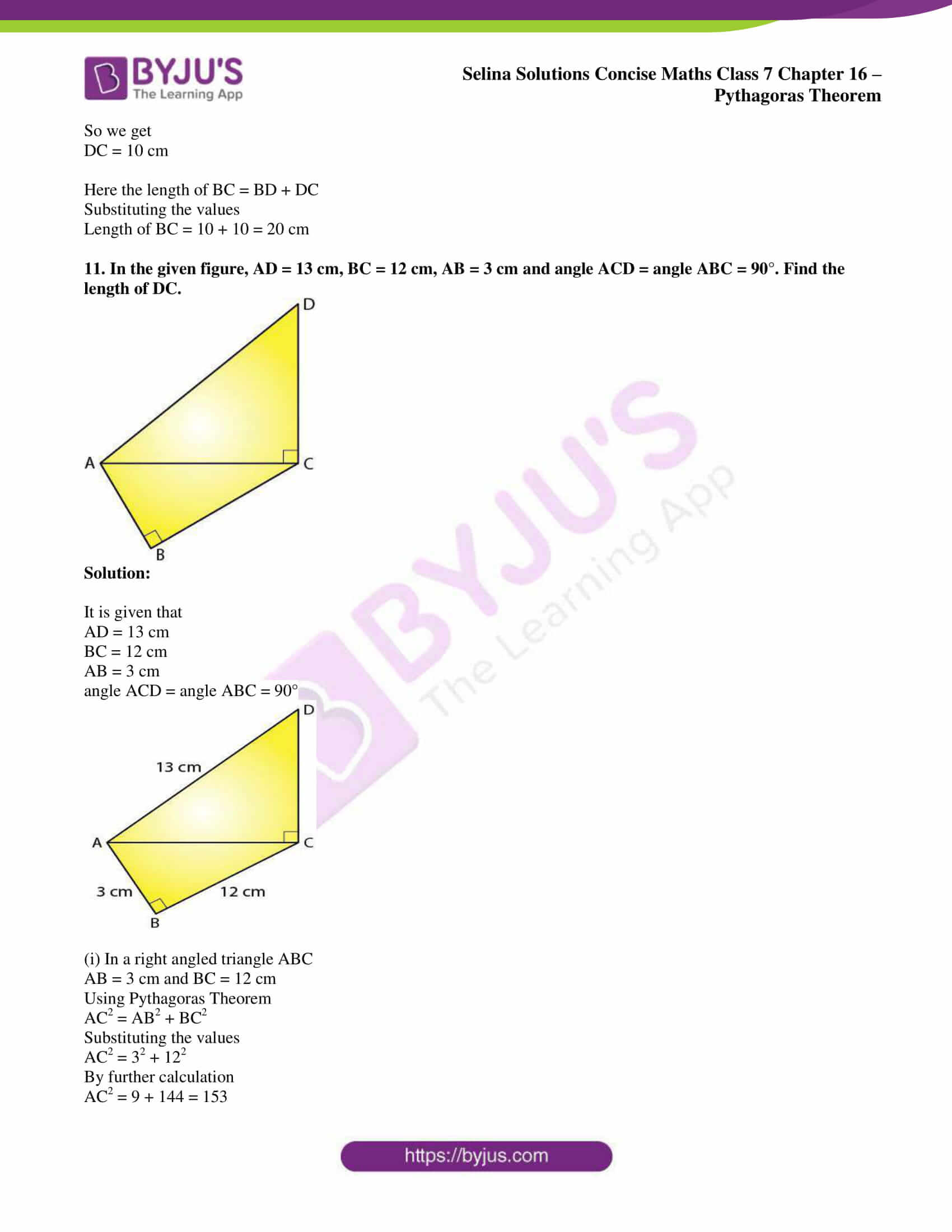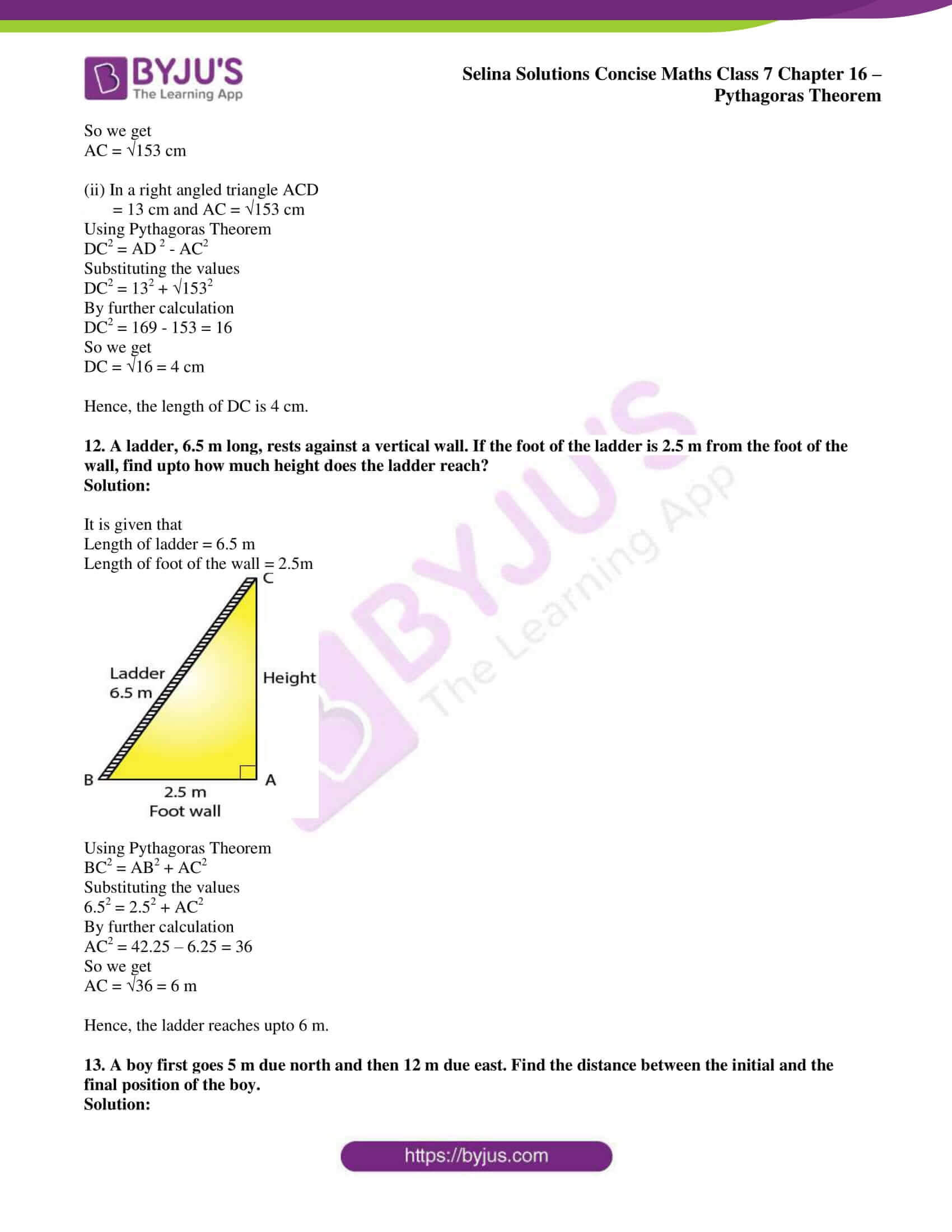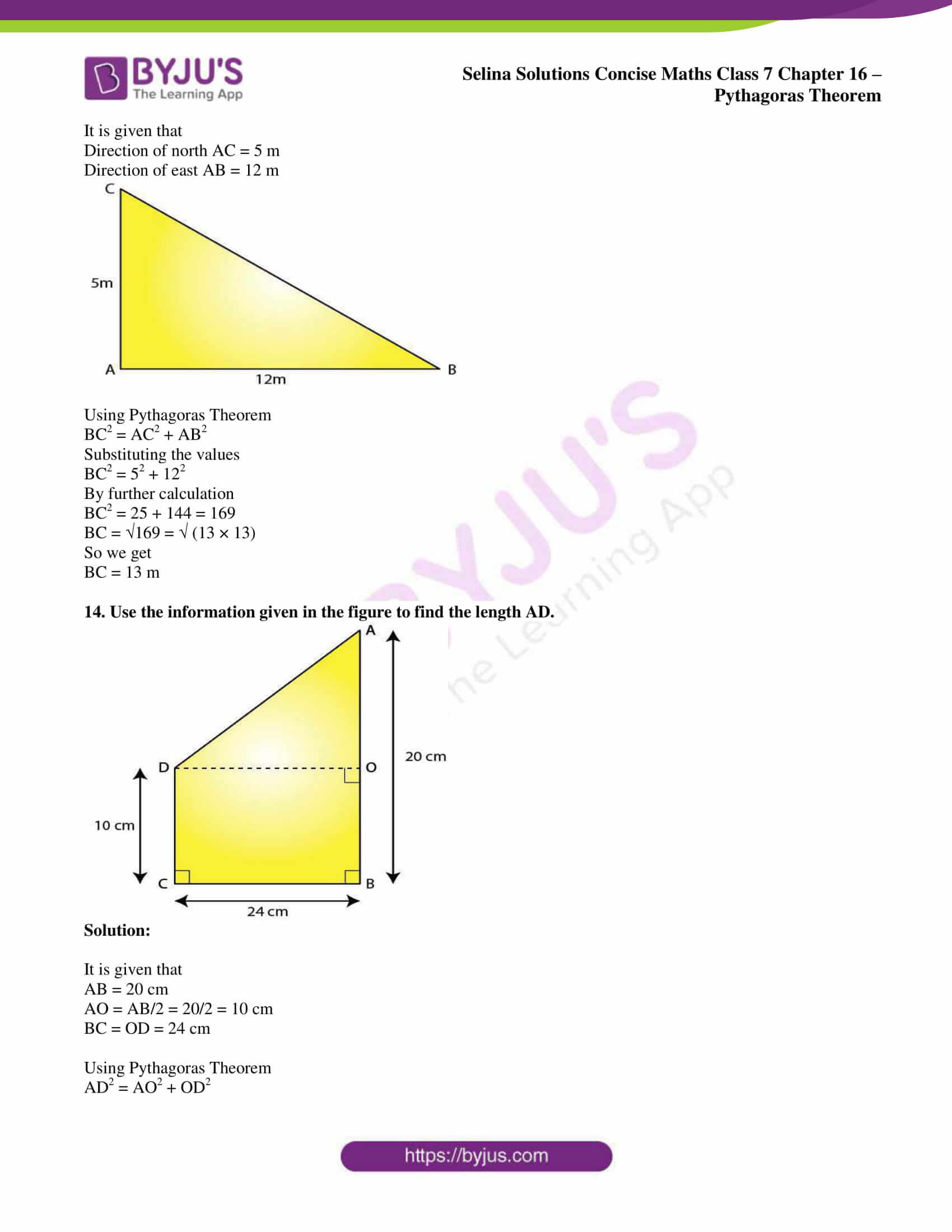## Access Selina Solutions Concise Maths Class 7 Chapter 16: Pythagoras Theorem

Exercise 16 page: 189

1. Triangle ABC is right-angled at vertex A. Calculate the length of BC, if AB = 18 cm and AC = 24 cm.

Solution:

It is given that

Triangle ABC is right-angled at vertex A

AB = 18 cm and AC = 24 cm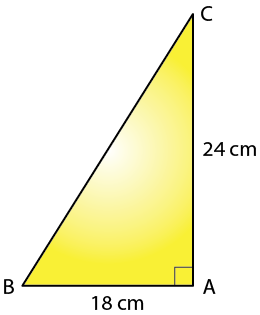Using Pythagoras Theorem

BC2 = AB2 + AC2

Substituting the values

BC2 = 182 + 242

By further calculation

BC2 = 324 + 576 = 900

BC = √900 = √ (30 × 30)

So we get

BC = 30 cm

2. Triangle XYZ is right-angled at vertex Z. Calculate the length of YZ, if XY = 13 cm and XZ = 12 cm.

Solution:

It is given that

Triangle XYZ is right-angled at vertex Z

XY = 13 cm and XZ = 12 cm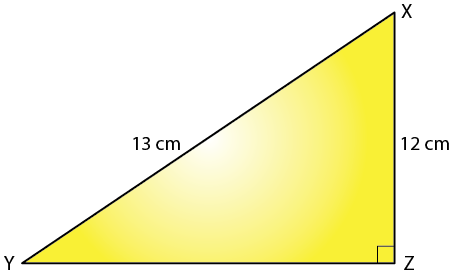Using Pythagoras Theorem

XY2 = XZ2 + YZ2

Substituting the values

132 = 122 + YZ2

By further calculation

YZ2 = 132 – 122

YZ2 = 169 – 144 = 25

YZ = √25 = √ (5 × 5)

So we get

YZ = 5 cm

3. Triangle PQR is right-angled at vertex R. Calculate the length of PR, if:
PQ = 34 cm and QR = 33.6 cm.

Solution:

It is given that

Triangle PQR is right-angled at vertex R

PQ = 34 cm and QR = 33.6 cm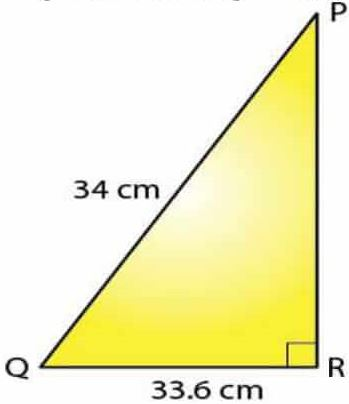Using Pythagoras Theorem

PQ2 = PR2 + QR2

Substituting the values

342 = PR2 + 33.62

By further calculation

1156 = PR2 + 1128.96

PR2 = 1156 – 1128.96

PR = √27.04

So we get

PR = 5.2 cm

4. The sides of a certain triangle are given below. Find, which of them is right-triangle

(i) 16 cm, 20 cm and 12 cm

(ii) 6 m, 9 m and 13 m

Solution:

(i) 16 cm, 20 cm and 12 cm
The triangle will be right angled if square of the largest side is equal to the sum of the squares of the other two sides.

Here we have 202, 162 and 122

We can write it as

202 = 162 + 122

By further calculation

400 = 256 + 144

So we get

400 = 400

Hence, the given triangle is right angled.

(ii) 6 m, 9 m and 13 m

The triangle will be right angled if square of the largest side is equal to the sum of the squares of the other two sides.

Here 132 = 92 + 62

By further calculation

169 = 81 + 36

So we get

169 ≠ 117

Hence, the given triangle is not right angled.

5. In the given figure, angle BAC = 90°, AC = 400 m and AB = 300 m. Find the length of BC.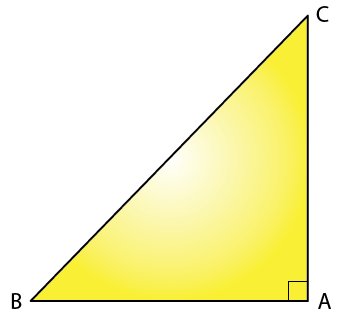Solution:

It is given that

BAC = 90°, AC = 400 m and AB = 300 m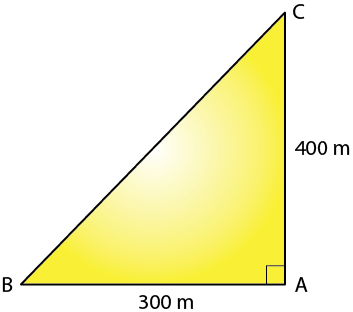Using Pythagoras Theorem

BC2 = AB2 + AC2

Substituting the values

BC2 = 3002 + 4002

By further calculation

BC2 = 90000 + 160000 = 250000

BC = √250000

So we get

BC = 500 m

6. In the given figure, angle ACP = ∠BDP = 90°, AC = 12 m, BD = 9 m and PA= PB = 15 m. Find:
(i) CP
(ii) PD
(iii) CD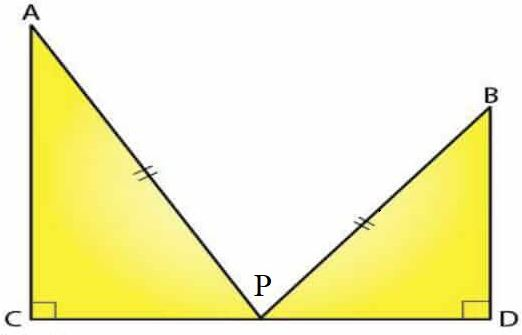Solution:

It is given that

ACP = ∠BDP = 90°

AC = 12 m

BD = 9 m

PA= PB = 15 m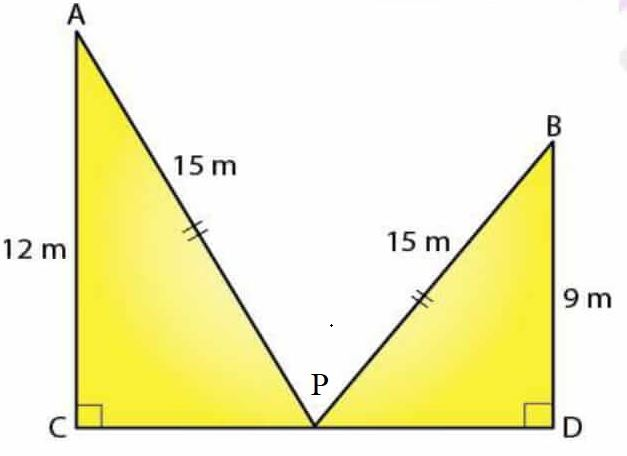(i) In the right angled triangle ACP

AP2 = AC2 + CP2

Substituting the values

152 = 122 + CP2

By further calculation

225 = 144 + CP2

CP2 = 225 – 144 = 81

So we get

CP = √81 = 9 m

(ii) In the right angled triangle BPD

PB2 = BD2 + PD2

Substituting the values

152 = 92 + PD2

By further calculation

225 = 81 + PD2

PD2 = 225 – 81 = 144

So we get

PD = √144 = 12 m

(iii) We know that

CP = 9 m

PD = 12 m

So we get

CD = CP + PD

Substituting the values

CD = 9 + 12 = 21 m

7. In triangle PQR, angle Q = 90°, find:
(i) PR, if PQ = 8 cm and QR = 6 cm
(ii) PQ, if PR = 34 cm and QR = 30 cm

Solution:

(i) It is given that

PQ = 8 cm and QR = 6 cm

∠PQR = 900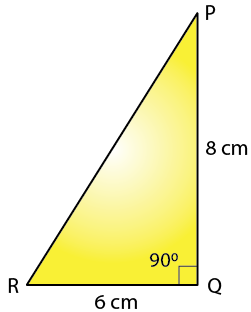Using Pythagoras Theorem

PR2 = PQ2 + QR2

Substituting the values

PR2 = 82 + 62

By further calculation

PR2 = 64 + 36 = 100

PR = √100

So we get

PR = 10 cm

(ii) It is given that

PR = 34 cm and QR = 30 cm

∠PQR = 900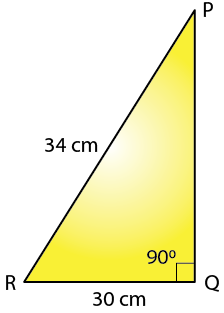Using Pythagoras Theorem

PR2 = PQ2 + QR2

Substituting the values

342 = PQ2 + 302

By further calculation

1156 = PQ2 + 900

PQ2 = 1156 – 900 = 256

PQ = √256

So we get

PQ = 16 cm

8. Show that the triangle ABC is a right-angled triangle; if:

AB = 9 cm, BC = 40 cm and AC = 41 cm

Solution:

It is given that

AB = 9 cm

BC = 40 cm

AC = 41 cm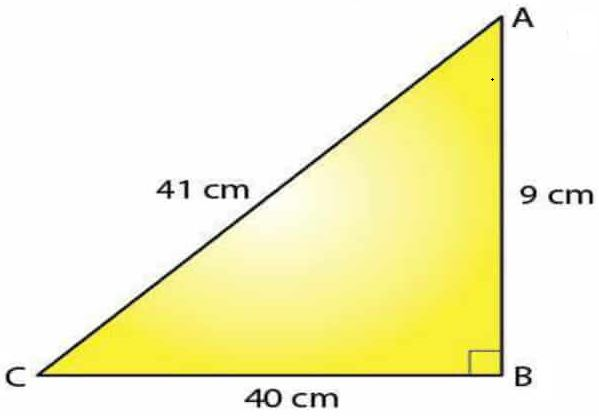The triangle will be right angled if square of the largest side is equal to the sum of the squares of the other two sides.

Using Pythagoras Theorem

AC2 = BC2 + AB2

Substituting the values

412 = 402 + 92

By further calculation

1681 = 1600 + 81

So we get

1681 = 1681

Therefore, ABC is a right-angled triangle.

9. In the given figure, angle ACB = 90° = angle ACD. If AB = 10 cm, BC = 6 cm and AD = 17 cm, find:

(i) AC

(ii) CD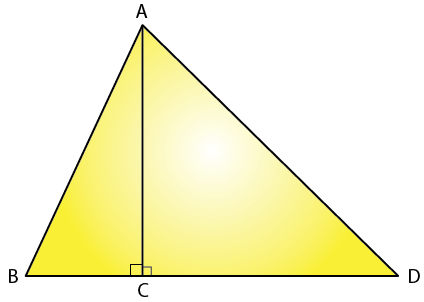Solution:

It is given that

angle ACB = 90° = angle ACD

AB = 10 cm, BC = 6 cm and AD = 17 cm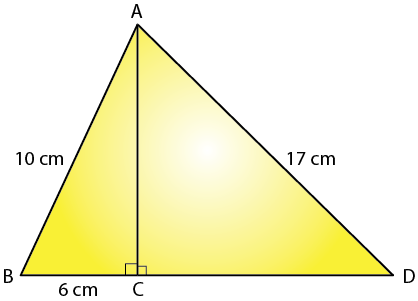(i) In the right angled triangle ABC

BC = 6 cm and AB = 10cm

Using Pythagoras Theorem

AB2 = AC2 + BC2

Substituting the values

102 = AC2 + 62

By further calculation

100 = AC2 + 36

AC2 = 100 – 36 = 64

AC = √64 = √ (8 × 8)

So we get

AC = 8 cm

(ii) In the right angled triangle ACD

AD = 17 cm and AC = 8cm

Using Pythagoras Theorem

Substituting the values

172 = 82 + CD2

By further calculation

289 = 64 + CD2

CD2 = 289 – 64 = 225

CD = √225 = √ (15 × 15)

So we get

CD = 15 cm

10. In the given figure, angle ADB = 90°, AC = AB = 26 cm and BD = DC. If the length of AD = 24 cm; find the length of BC.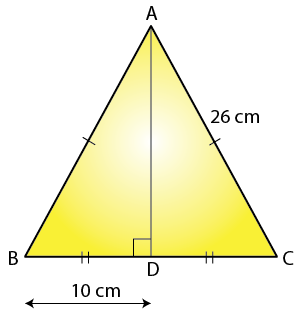Solution:

It is given that

AC = AB = 26 cm

BD = DC

Using Pythagoras Theorem

Substituting the values

262 = 242 + DC2

By further calculation

676 = 576 + DC2

DC2 = 676 – 576 = 100

DC = √100

So we get

DC = 10 cm

Here the length of BC = BD + DC

Substituting the values

Length of BC = 10 + 10 = 20 cm

11. In the given figure, AD = 13 cm, BC = 12 cm, AB = 3 cm and angle ACD = angle ABC = 90°. Find the length of DC.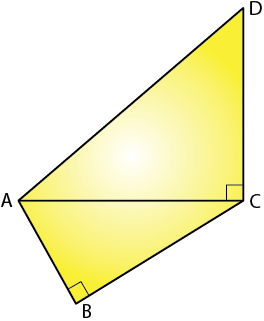Solution:

It is given that

BC = 12 cm

AB = 3 cm

angle ACD = angle ABC = 90°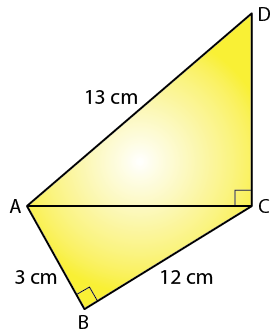(i) In a right angled triangle ABC

AB = 3 cm and BC = 12 cm

Using Pythagoras Theorem

AC2 = AB2 + BC2

Substituting the values

AC2 = 32 + 122

By further calculation

AC2 = 9 + 144 = 153

So we get

AC = √153 cm

(ii) In a right angled triangle ACD

AD = 13 cm and AC = √153 cm

Using Pythagoras Theorem

Substituting the values

DC2 = 132 + √1532

By further calculation

DC2 = 169 – 153 = 16

So we get

DC = √16 = 4 cm

Hence, the length of DC is 4 cm.

12. A ladder, 6.5 m long, rests against a vertical wall. If the foot of the ladder is 2.5 m from the foot of the wall, find upto how much height does the ladder reach?

Solution:

It is given that

Length of ladder = 6.5 m

Length of foot of the wall = 2.5m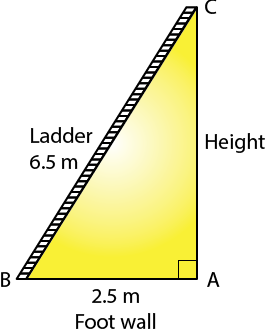Using Pythagoras Theorem

BC2 = AB2 + AC2

Substituting the values

6.52 = 2.52 + AC2

By further calculation

AC2 = 42.25 – 6.25 = 36

So we get

AC = √36 = 6 m

Hence, the ladder reaches upto 6 m.

13. A boy first goes 5 m due north and then 12 m due east. Find the distance between the initial and the final position of the boy.

Solution:

It is given that

Direction of north AC = 5 m

Direction of east AB = 12 m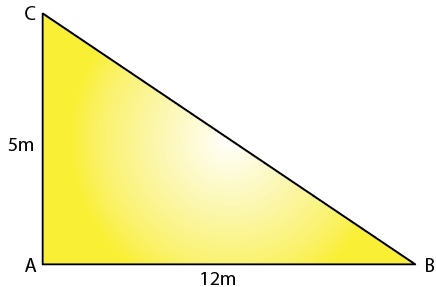Using Pythagoras Theorem

BC2 = AC2 + AB2

Substituting the values

BC2 = 52 + 122

By further calculation

BC2 = 25 + 144 = 169

BC = √169 = √ (13 × 13)

So we get

BC = 13 m

14. Use the information given in the figure to find the length AD.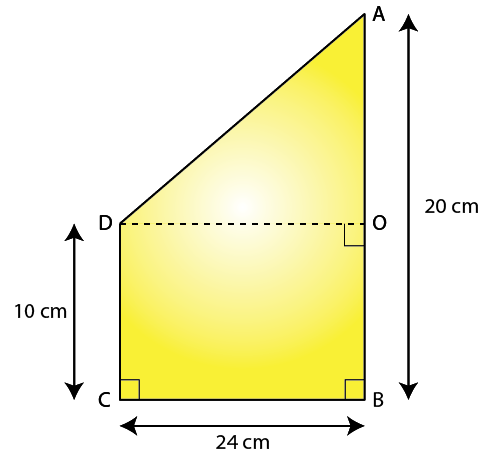Solution:

It is given that

AB = 20 cm

AO = AB/2 = 20/2 = 10 cm

BC = OD = 24 cm

Using Pythagoras Theorem

Substituting the values